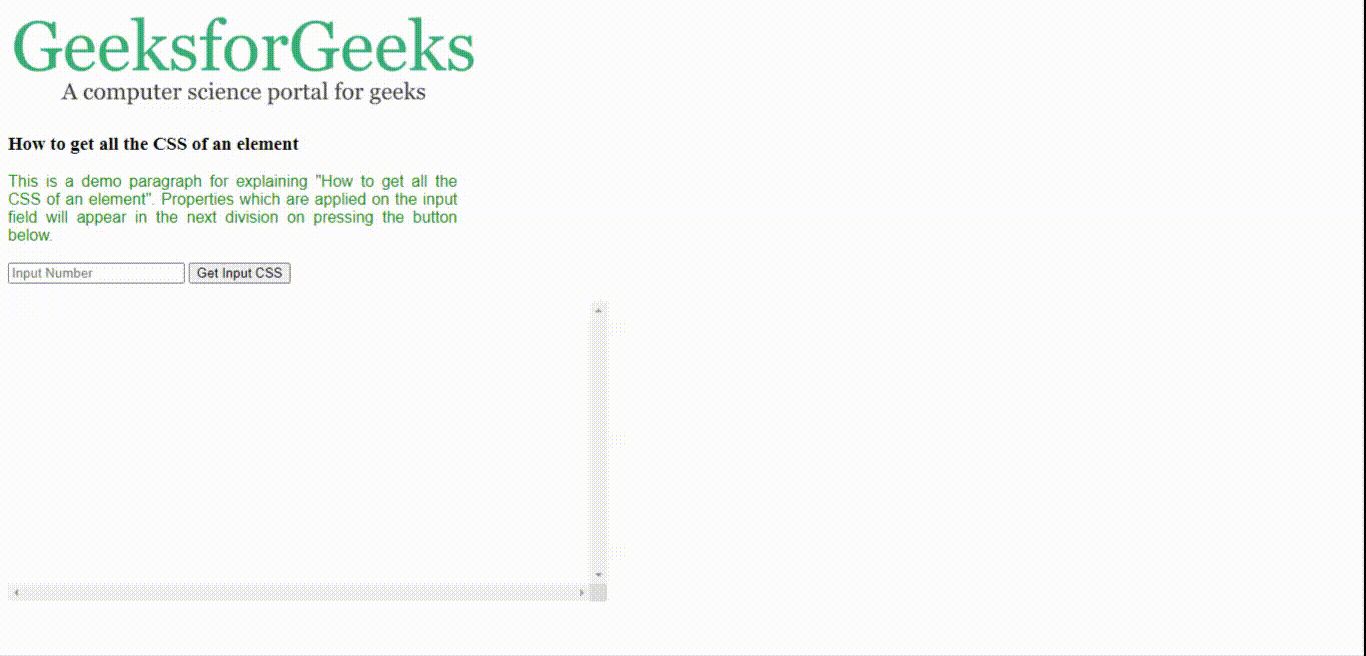# How to get all CSS styles that are applied directly to an element using JavaScript ?

The CSS of an element can be obtained using the getComputedStyle element function in JavaScript. It returns a JavaScript object containing CSS properties and their values. This object is indexed and iterable over the property names. The getPropertyValue(property) is used to get the value of a property.

In the below code, passing your element object to the getCSS( element ) gets all the properties using getComputedStyle(element) and getPropertyValue(property) and write them in a separate division. You can scroll the results to see all the properties.

Example 1: The following example demonstrates getting all properties of <div> element with id=’srcDiv’.## HTML

 ` ` `<``html``> ` ` `  `<``head``> ` `    ``<``style``> ` `        ``#srcDiv { ` `            ``width: 450px; ` `            ``font-family: sans-serif; ` `            ``text-align: justify; ` `            ``justify-content: center; ` `            ``color: green; ` `        ``} ` ` `  `        ``#resDiv { ` `            ``height: 300px; ` `            ``overflow: scroll; ` `        ``} ` `    `` ` ` `  `    ``<``script``> ` `        ``function getCSS(element) { ` `            ``var css_data = ''; ` `            ``var css_obj = getComputedStyle(element); ` ` `  `            ``for (var i = 0; i < ``css_obj.length``; i++) { ` `                ``css_data += ` `                    ``css_obj[i] + ':' +  ` `                    ``css_obj.getPropertyValue(css_obj[i]) ` `                    ``+ ';
'; ` `            ``} ` `            ``document.getElementById('resDiv') ` `                    ``.innerHTML = css_data; ` `            ``return; ` `        ``} ` `    `` ` ` ` ` `  `<``body``> ` `    ``<``img` `src``=``"logo.png"` `/><``br` `/> ` ` `  `    ``<``h3``> ` `        ``How to get all the  ` `        ``CSS of an element ` `    `` ` `    ``<``br` `/> ` `     `  `    ``<``div` `id``=``"srcDiv"``> ` `        ``This is a demo paragraph for  ` `        ``explaining "How to get all the  ` `        ``CSS of an element". Properties  ` `        ``which are applied on this  ` `        ``division will appear in the ` `        ``next division on pressing the  ` `        ``button below. ` `    `` ` `    ``<``br` `/><``br` `/> ` ` `  `    ``<``button` `onclick="getCSS(document ` `        ``.getElementById('srcDiv'))"> ` `        ``Get CSS ` `    `` ` `    ``<``br` `/><``br` `/> ` ` `  `    ``<``div` `id``=``"resDiv"``> ` ` ` ` `  ` `

Output: On clicking the ‘Get CSS’ button, the output shows.Example 2: Below is an example to obtain the property of an <input> element with id=’inputTxt’.## HTML

 ` ` `<``html``> ` ` `  `<``head``> ` `    ``<``style``> ` `        ``#srcDiv { ` `            ``width: 450px; ` `            ``font-family: sans-serif; ` `            ``text-align: justify; ` `            ``justify-content: center; ` `            ``color: green; ` `        ``} ` ` `  `        ``#inputTxt { ` `            ``color: red; ` `        ``} ` ` `  `        ``#resDiv { ` `            ``height: 300px; ` `            ``width: 600px; ` `            ``overflow: scroll; ` `        ``} ` `    `` ` ` `  `    ``<``script``> ` `        ``function getCSS(element) { ` `            ``var css_data = ''; ` `            ``var css_obj = getComputedStyle(element); ` `            ``for (var i = 0; i < ``css_obj.length``; i++) { ` `                ``css_data += ` `                    ``css_obj[i] + ':' + css_obj ` `                        ``.getPropertyValue(css_obj[i]) ` `                        ``+ ';
'; ` `            ``} ` `            ``document.getElementById('resDiv') ` `                    ``.innerHTML = css_data; ` `            ``return; ` `        ``} ` `    `` ` ` ` ` `  `<``body``> ` `    ``<``img` `src``=``"logo.png"` `/><``br` `/> ` ` `  `    ``<``h3``> ` `        ``How to get all the  ` `        ``CSS of an element ` `    `` ` ` `  `    ``<``div` `id``=``"srcDiv"``> ` `        ``This is a demo paragraph for explaining  ` `        ``"How to get all the CSS of an element".  ` `        ``Properties which are applied on the input  ` `        ``field will appear in the next division on ` `        ``pressing the button below. ` `    `` ` `    ``<``br` `/> ` ` `  `    ``<``input` `id``=``"inputTxt"` `type``=``"number"`  `            ``placeholder``=``"Input Number"` `/> ` ` `  `    ``<``button` `onclick="getCSS(document ` `        ``.getElementById('inputTxt'))"> ` `        ``Get Input CSS ` `    `` ` `    ``<``br` `/><``br` `/> ` `     `  `    ``<``div` `id``=``"resDiv"``> ` ` ` ` `  ` `

Output: On clicking the ‘Get Input CSS’ button, the following output shows.Note: Please note that the object being passed into the getCSS() function in each example.My Personal Notes arrow_drop_upCheck out this Author's contributed articles.

If you like GeeksforGeeks and would like to contribute, you can also write an article using contribute.geeksforgeeks.org or mail your article to contribute@geeksforgeeks.org. See your article appearing on the GeeksforGeeks main page and help other Geeks.

Please Improve this article if you find anything incorrect by clicking on the "Improve Article" button below.

Article Tags :
Practice Tags :

Be the First to upvote.

Please write to us at contribute@geeksforgeeks.org to report any issue with the above content.